﻿ Twofish Properties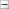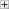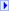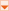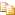The Twofish type exposes the following members.

#Properties

NameDescription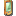BlockSize
Gets or sets the block size, in bits, of the cryptographic operation.
(Inherited from SymmetricAlgorithm.)FeedbackSize
Gets or sets the feedback size, in bits, of the cryptographic operation.
(Inherited from SymmetricAlgorithm.)IV
Gets or sets the initialization vector (IV) for the symmetric algorithm.
(Inherited from SymmetricAlgorithm.)Key
Gets or sets the secret key for the symmetric algorithm.
(Inherited from SymmetricAlgorithm.)KeySize
Gets or sets the size, in bits, of the secret key used by the symmetric algorithm.
(Inherited from SymmetricAlgorithm.)LegalBlockSizes
Gets the block sizes that are supported by the symmetric algorithm.
(Inherited from SymmetricAlgorithm.)LegalKeySizes
Gets the key sizes that are supported by the symmetric algorithm.
(Inherited from SymmetricAlgorithm.)Mode
Gets or sets the mode for operation of the symmetric algorithm.
(Inherited from SymmetricAlgorithm.)Padding
Gets or sets the padding mode used in the symmetric algorithm.
(Inherited from SymmetricAlgorithm.)